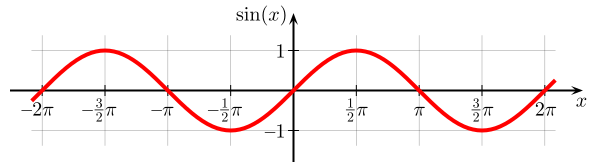## Position, Velocity, and Acceleration as a Function of Time

A wave is an oscillation that travels through space, accompanied by a transfer of energy.

### Learning Objectives

Contrast mechanical and electromagnetic waves

### Key Takeaways

#### Key Points

• The wave equation requires that the second time derivative of the waveform be proportional to its second spatial derivative.
• Waveforms describe the shape of physical waves, and can take the form of any function that repeats in space.
• One of the most common waveforms in physics is the sinusoid. Since any arbitray waveform can be generated by adding a set of sine waves, the physics governing a wave of an arbitrary shape can be described by using its sine wave components.

#### Key Terms

• waveform: The shape of a physical wave, such as sound or electromagnetic radiation. The shape can be any function that repeats in space.
• Fourier analysis: The study of the way general functions may be represented or approximated by sums of simpler trigonometric functions.

### Overview

A wave is an oscillation that travels through space, accompanied by a transfer of energy. Wave motion transfers energy from one point to another, often with no permanent displacement of the particles of the medium —that is, with little or no associated mass transport. They consist, instead, of oscillations or vibrations around almost fixed locations. There are two main types of waves. Mechanical waves propagate through a medium, and the substance of this medium is deformed. The deformation reverses itself owing to restoring forces resulting from its deformation.

The second main type of wave, electromagnetic waves, do not require a medium (although they may still propagate through a medium). Instead, they consist of periodic oscillations in electrical and magnetic fields generated by charged particles, and can therefore travel through a vacuum.

### Wave Equation

The shape of a wave can take the form of any function that repeats itself over some characteristic spatial scale $\lambda$, the wavelength (see ). More generally, waveforms are scalar functions $\text{u}$ which satisfy the wave equation, $\frac{\partial^2\text{u}}{\partial \text{t}^2}=\text{c}^2\nabla^2\text{u}$. This equation simply states that the acceleration of the waveform (Left: second derivative with respect to time) is proportional to the Laplacian (Right: second spatial derivative) of the same waveform. The constant of proportionality, $\text{c}^2$, is the square of the propagation speed of the wave.Common waveforms: A sample of several common, simple waveforms. A waveform is a function that repeats in space.

### Sine WavePlot of Sine: The sine function graphed on the Cartesian plane. In this graph, the angle x is given in radians (π = 180°).

Consider one of the most common waveforms, the sinusoid. A general form of a sinusoidal wave is $\text{y}(\text{x},\text{t}) = \text{A} \text{sin}(\text{kx}-\omega \text{t} + \phi)$, where A is the amplitude of the wave, $\omega$ is the wave’s angular frequency, k is the wavenumber, and $\phi$ is the phase of the sine wave given in radians. This waveform gives the displacement position (“y”) of a particle in a medium from its equilibrium as a function of both position “x” and time “t”.

By taking derivatives, it is evident that the wave equation given above holds for $\text{c} = \frac{\omega}{\text{k}}$, which is also called the phase speed of the wave. To find the velocity of a particle in the medium at x and t, we take the temporal derivative of the waveform to get $\frac{\partial \text{y}(\text{x},\text{t})}{\partial \text{t}} = -\text{A} \omega \text{cos}(\text{kx} - \omega \text{t} + \phi)$. Likewise, to find the acceleration of the displaced particle in the medium at x and t, we take the second derivative to get $\frac{\partial^2 \text{y}(\text{x},\text{t})}{\partial \text{t}^2} = - \text{A} \omega^2 \text{sin}(\text{kx} - \omega \text{t} + \phi)$. Note the phase relationship among the trigonometric functions in y(x,t), y'(x,t), y”(x,t). When the particle displacement is maximum or minimum, the velocity is 0. When the displacement is 0, particle velocity is either maximum or minimum. Similarly, the particle acceleration is maximum (or minimum) when the particle displacement is minimum (or maximum), respectively.

### Arbitrary Wave

We looked closely into the sinusoidal wave. But how about waves that has a general form? One important aspect of the wave equation is its linearity: the wave equation is linear in u and it is left unaltered by translations in space and time. Since a wave with an arbitrary shape can be represented by a sum of many sinusoidal waves (this is called Fourier analysis), we can generate a great variety of solutions of the wave equation by translating and summing sine waves that we just looked closely into.